GFG App
Open AppBrowser
Continue

# Three-dimensional Plotting in Python using Matplotlib

3D plots are very important tools for visualizing data that have three dimensions such as data that have two dependent and one independent variable. By plotting data in 3d plots we can get a deeper understanding of data that have three variables. We can use various matplotlib library functions to plot 3D plots.

## Example Of Three-dimensional Plotting using Matplotlib

We will first start with plotting the 3D axis using the Matplotlib library. For plotting the 3D axis we just have to change the projection parameter of plt.axes() from None to 3D.

## Python3

 `import` `numpy as np` `import` `matplotlib.pyplot as plt`     `fig ``=` `plt.figure()` `ax ``=` `plt.axes(projection``=``'3d'``)`

Output: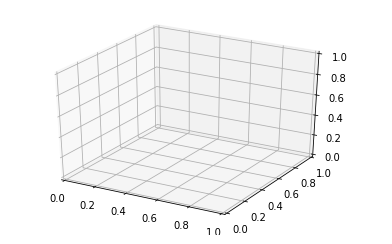Plotting 3D axes using matplotlib

With the above syntax three -dimensional axes are enabled and data can be plotted in 3 dimensions. 3 dimension graph gives a dynamic approach and makes data more interactive. Like 2-D graphs, we can use different ways to represent to plot 3-D graphs. We can make a scatter plot, contour plot, surface plot, etc. Let’s have a look at different 3-D plots.
Graphs with lines and points are the simplest 3-dimensional graph.  We will use ax.plot3d and ax.scatter functions to plot line and point graph respectively.

### 3-Dimensional Line Graph Using Matplotlib

For plotting the 3-Dimensional line graph we will use the mplot3d function from the mpl_toolkits library. For plotting lines in 3D we will have to initialize three variable points for the line equation. In our case, we will define three variables as x, y, and z.

## Python3

 `# importing mplot3d toolkits, numpy and matplotlib` `from` `mpl_toolkits ``import` `mplot3d` `import` `numpy as np` `import` `matplotlib.pyplot as plt`   `fig ``=` `plt.figure()`   `# syntax for 3-D projection` `ax ``=` `plt.axes(projection ``=``'3d'``)`   `# defining all 3 axis` `z ``=` `np.linspace(``0``, ``1``, ``100``)` `x ``=` `z ``*` `np.sin(``25` `*` `z)` `y ``=` `z ``*` `np.cos(``25` `*` `z)`   `# plotting` `ax.plot3D(x, y, z, ``'green'``)` `ax.set_title(``'3D line plot geeks for geeks'``)` `plt.show()`

Output: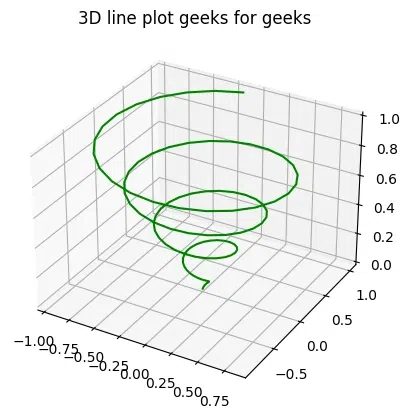3D line plot graph using the matplotlib library

### 3-Dimensional Scattered Graph Using Matplotlib

To plot the same graph using scatter points we will use the scatter() function from matplotlib. It will plot the same line equation using distinct points.

## Python3

 `# importing mplot3d toolkits` `from` `mpl_toolkits ``import` `mplot3d` `import` `numpy as np` `import` `matplotlib.pyplot as plt`   `fig ``=` `plt.figure()`   `# syntax for 3-D projection` `ax ``=` `plt.axes(projection ``=``'3d'``)`   `# defining axes` `z ``=` `np.linspace(``0``, ``1``, ``100``)` `x ``=` `z ``*` `np.sin(``25` `*` `z)` `y ``=` `z ``*` `np.cos(``25` `*` `z)` `c ``=` `x ``+` `y` `ax.scatter(x, y, z, c ``=` `c)`   `# syntax for plotting` `ax.set_title(``'3d Scatter plot geeks for geeks'``)` `plt.show()`

Output: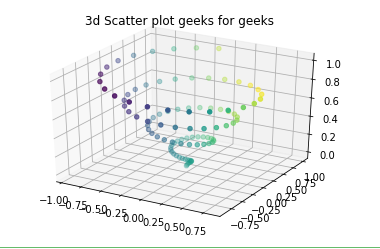3D point plot using Matplotlib library

### Surface Graphs using Matplotlib library

Surface graphs and Wireframes graph work on gridded data. They take the grid value and plot it on a three-dimensional surface. We will use the plot_surface() function to plot the surface plot.

## Python3

 `# importing libraries` `from` `mpl_toolkits ``import` `mplot3d` `import` `numpy as np` `import` `matplotlib.pyplot as plt`   `# defining surface and axes` `x ``=` `np.outer(np.linspace(``-``2``, ``2``, ``10``), np.ones(``10``))` `y ``=` `x.copy().T` `z ``=` `np.cos(x ``*``*` `2` `+` `y ``*``*` `3``)`   `fig ``=` `plt.figure()`   `# syntax for 3-D plotting` `ax ``=` `plt.axes(projection``=``'3d'``)`   `# syntax for plotting` `ax.plot_surface(x, y, z, cmap``=``'viridis'``,\` `                ``edgecolor``=``'green'``)` `ax.set_title(``'Surface plot geeks for geeks'``)` `plt.show()`

Output: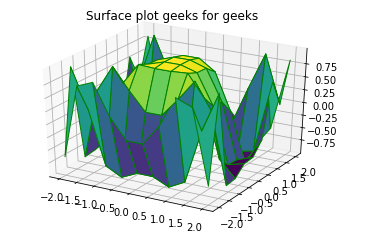Surface plot using matplotlib library

### Wireframes graph using Matplotlib library

For plotting the wireframes graph we will use the plot_wireframe() function from the matplotlib library.

## Python3

 `from` `mpl_toolkits ``import` `mplot3d` `import` `numpy as np` `import` `matplotlib.pyplot as plt`     `# function for z axis` `def` `f(x, y):` `    ``return` `np.sin(np.sqrt(x ``*``*` `2` `+` `y ``*``*` `2``))`   `# x and y axis` `x ``=` `np.linspace(``-``1``, ``5``, ``10``)` `y ``=` `np.linspace(``-``1``, ``5``, ``10``)` ` `  `X, Y ``=` `np.meshgrid(x, y)` `Z ``=` `f(X, Y)`   `fig ``=` `plt.figure()` `ax ``=` `plt.axes(projection ``=``'3d'``)` `ax.plot_wireframe(X, Y, Z, color ``=``'green'``)` `ax.set_title(``'wireframe geeks for geeks'``);`

Output: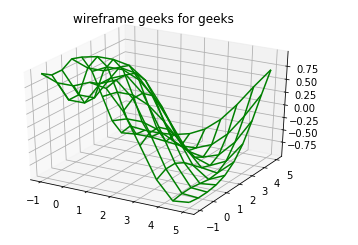3D wireframe graph using the matplotlib library

### Contour Graphs using Matplotlib library

The contour graph takes all the input data in two-dimensional regular grids, and the Z data is evaluated at every point. We use the ax.contour3D function to plot a contour graph. Contour plots are an excellent way to visualize optimization plots.

## Python3

 `def` `function(x, y):` `    ``return` `np.sin(np.sqrt(x ``*``*` `2` `+` `y ``*``*` `2``))`     `x ``=` `np.linspace(``-``10``, ``10``, ``40``)` `y ``=` `np.linspace(``-``10``, ``10``, ``40``)`   `X, Y ``=` `np.meshgrid(x, y)` `Z ``=` `function(X, Y)`   `fig ``=` `plt.figure(figsize``=``(``10``, ``8``))` `ax ``=` `plt.axes(projection``=``'3d'``)`   `ax.plot_surface(X, Y, Z, cmap``=``'cool'``, alpha``=``0.8``)`   `ax.set_title('``3D` `Contour Plot of function(x, y) ``=``\` `                ``sin(sqrt(x^``2` `+` `y^``2``))', fontsize``=``14``)` `ax.set_xlabel(``'x'``, fontsize``=``12``)` `ax.set_ylabel(``'y'``, fontsize``=``12``)` `ax.set_zlabel(``'z'``, fontsize``=``12``)`   `plt.show()`

Output: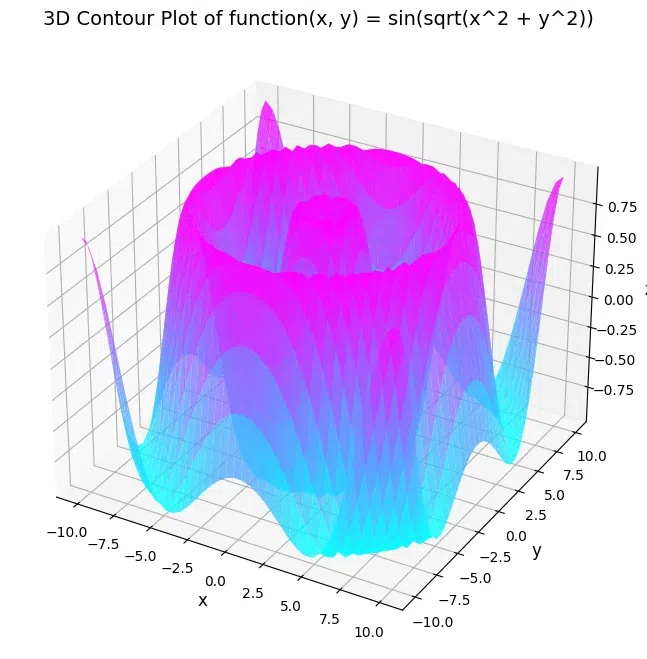3D contour plot of a function using matplotlib

### Plotting Surface Triangulations In Python

The above graph is sometimes overly restricted and inconvenient. So by this method, we use a set of random draws. The function ax.plot_trisurf is used to draw this graph. It is not that clear but more flexible.

## Python3

 `import` `numpy as np` `import` `matplotlib.pyplot as plt` `from` `mpl_toolkits.mplot3d ``import` `Axes3D` `from` `matplotlib.tri ``import` `Triangulation`   `def` `f(x, y):` `    ``return` `np.sin(np.sqrt(x ``*``*` `2` `+` `y ``*``*` `2``))`   `x ``=` `np.linspace(``-``6``, ``6``, ``30``)` `y ``=` `np.linspace(``-``6``, ``6``, ``30``)` `X, Y ``=` `np.meshgrid(x, y)` `Z ``=` `f(X, Y)`   `tri ``=` `Triangulation(X.ravel(), Y.ravel())`   `fig ``=` `plt.figure(figsize``=``(``10``, ``8``))` `ax ``=` `fig.add_subplot(``111``, projection``=``'3d'``)`   `ax.plot_trisurf(tri, Z.ravel(), cmap``=``'cool'``, edgecolor``=``'none'``, alpha``=``0.8``)`   `ax.set_title('Surface Triangulation Plot of f(x, y) ``=``\` `                ``sin(sqrt(x^``2` `+` `y^``2``))', fontsize``=``14``)` `ax.set_xlabel(``'x'``, fontsize``=``12``)` `ax.set_ylabel(``'y'``, fontsize``=``12``)` `ax.set_zlabel(``'z'``, fontsize``=``12``)`   `plt.show()`

Output: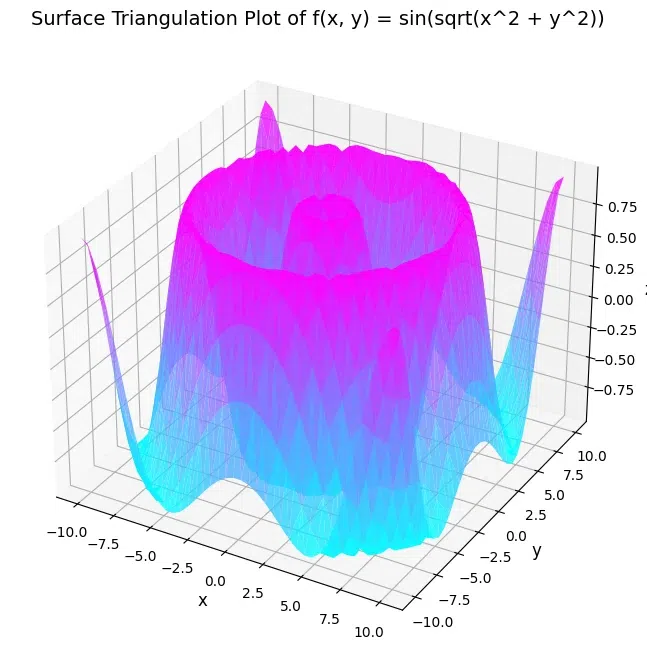Surface triangulation graph of  a contour plot using matplotlib

### Plotting Möbius strip In Python

Möbius strip also called the twisted cylinder, is a one-sided surface without boundaries. To create the Möbius strip think about its parameterization, it’s a two-dimensional strip, and we need two intrinsic dimensions. Its angle range from 0 to 2 pie around the loop and its width ranges from -1 to 1.

## Python3

 `import` `numpy as np` `import` `matplotlib.pyplot as plt` `from` `mpl_toolkits.mplot3d ``import` `Axes3D`   `# Define the parameters of the Möbius strip` `R ``=` `2`   `# Define the Möbius strip surface` `u ``=` `np.linspace(``0``, ``2``*``np.pi, ``100``)` `v ``=` `np.linspace(``-``1``, ``1``, ``100``)` `u, v ``=` `np.meshgrid(u, v)` `x ``=` `(R ``+` `v``*``np.cos(u``/``2``)) ``*` `np.cos(u)` `y ``=` `(R ``+` `v``*``np.cos(u``/``2``)) ``*` `np.sin(u)` `z ``=` `v ``*` `np.sin(u``/``2``)`   `# Create the plot` `fig ``=` `plt.figure()` `ax ``=` `fig.add_subplot(``111``, projection``=``'3d'``)`   `# Plot the Möbius strip surface` `ax.plot_surface(x, y, z, alpha``=``0.5``)`   `# Set plot properties` `ax.set_xlabel(``'X'``)` `ax.set_ylabel(``'Y'``)` `ax.set_zlabel(``'Z'``)` `ax.set_title(``'Möbius Strip'``)`   `# Set the limits of the plot` `ax.set_xlim([``-``3``, ``3``])` `ax.set_ylim([``-``3``, ``3``])` `ax.set_zlim([``-``3``, ``3``])`   `# Show the plot` `plt.show()`

Output: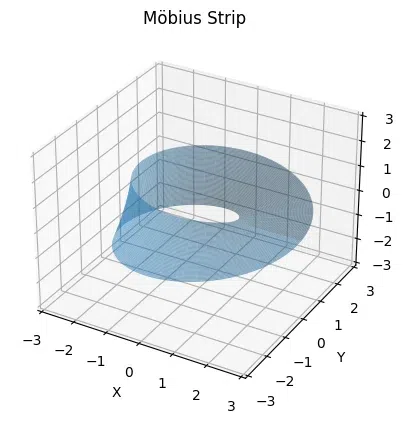Mobius strip plot using matplotlib library

My Personal Notes arrow_drop_up